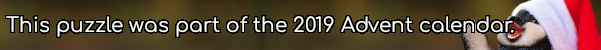mscroggs.co.uk
mscroggs.co.uksubscribe

# Puzzles

## 20 DecemberThe integers from 2 to 14 (including 2 and 14) are written on 13 cards (one number per card). You and a friend take it in turns to take one of the numbers.
When you have both taken five numbers, you notice that the product of the numbers you have collected is equal to the product of the numbers that your friend has collected. What is the product of the numbers on the three cards that neither of you has taken?

## 19 DecemberThe diagram below shows three squares and five circles. The four smaller circles are all the same size, and the red square's vertices are the centres of these circles.
The area of the blue square is 14 units. What is the area of the red square?

## 18 DecemberThe final round of game show starts with £1,000,000. You and your opponent take it in turn to take any value between £1 and £900. At the end of the round, whoever takes the final pound gets to take the money they have collected home, while the other player leaves with nothing.
You get to take an amount first. How much money should you take to be certain that you will not go home with nothing?
Tags: numbers, games

## 17 DecemberEve picks a three digit number then reverses its digits to make a second number. The second number is larger than her original number.
Eve adds her two numbers together; the result is 584. What was Eve's original number?

## 16 DecemberArrange the digits 1-9 in a 3×3 square so that: the median number in the first row is 6; the median number in the second row is 3; the mean of the numbers in the third row is 4; the mean of the numbers in the second column is 7; the range of the numbers in the third column is 2, The 3-digit number in the first column is today's number.
 median 6 median 3 mean 4 today's number mean 7 range 2

## 15 DecemberThere are 5 ways to make 30 by multiplying positive integers (including the trivial way):
• 30
• 2×15
• 3×10
• 5×6
• 2×3×5
Today's number is the number of ways of making 30030 by multiplying.

## 14 DecemberDuring one day, a digital clock shows times from 00:00 to 23:59. How many times during the day do the four digits shown on the clock add up to 14?

## 13 DecemberEach clue in this crossnumber (except 5A) gives a property of that answer that is true of no other answer. For example: 7A is a multiple of 13; but 1A, 3A, 5A, 1D, 2D, 4D, and 6D are all not multiples of 13. No number starts with 0.

## Archive

Show me a random puzzle
▼ show ▼